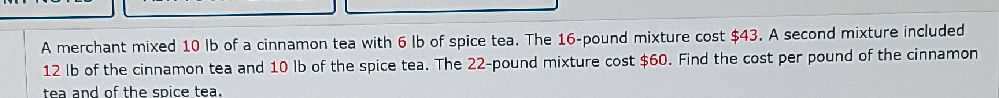### ¿Todavía tienes preguntas de matemáticas?

Pregunte a nuestros tutores expertos
Algebra
PreguntaA merchant mixed $$10$$ lb of a cinnamon tea with $$6 lb$$ of spice tea. The $$16$$ -pound mixture cost $$\ 43$$ . A second mixture included $$12 lb$$ of the cinnamon tea and $$10 lb$$ of the spice tea. The 22-pound mixture cost $$\ 60$$ . Find the cost per pound of the cinnamon tea and of the spice tea.